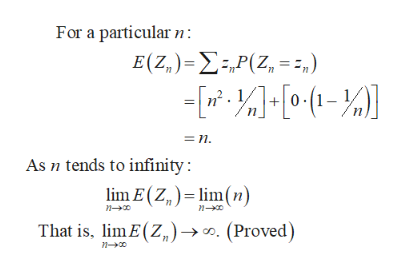# Let Z1,...,Zn be a sequence of random variables. For every n = 1, 2;..., P(Zn =n^2) = 1/n, and P(Zn = 0) = 1 - 1/n. In other words, Zn is binary. Show that(a) limit as n goes to infinity E(Zn)= infinity

Question
152 views

Let Z1,...,Zn be a sequence of random variables. For every n = 1, 2;..., P(Zn =
n^2) = 1/n, and P(Zn = 0) = 1 - 1/n. In other words, Zn is binary. Show that
(a) limit as n goes to infinity E(Zn)= infinity

check_circle

Step 1

Introduction:

For a particular value of n, P (Zn = n2) = 1/n and P (Zn = 0) = 1 – 1/n.

Step 2

Calculation:

The expectation, as n tends to infinity, ...help_outlineImage TranscriptioncloseFor a particular E(Ζ,) - Σ-Ρ(Ζ, = 3.) - 0- =n As n tends to infinity: lim E(Z,) lim(n) n0 n- That is. lm E(Z)». (Proved) fullscreen

### Want to see the full answer?

See Solution

#### Want to see this answer and more?

Solutions are written by subject experts who are available 24/7. Questions are typically answered within 1 hour.*

See Solution
*Response times may vary by subject and question.
Tagged in

### Statistics